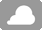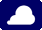3 ~ 10℃ 杭州天气

?• 商场
• 理发美容中心
• 收费停车场
• 前台贵重物品保险柜
• 中餐厅
• KTV
• 电梯
• 暖气
• 餐厅
• 无烟楼层
• 非经营性客人休息区
• 公共区域闭路电视监控系统
• 公共音响系统
• 礼品廊
• 行政楼层
• 多媒体演示系统
• 公用区wifi(免费)
• 公共区域禁烟
• 花园
• 旅游交通图
• 新风系统
• 保安人员
• 投影设备
• 自动售货机
• 自动售货机（饮品）
• 自动售货机（零食）
• 洗衣房

• 叫车服务
• 会议厅
• 洗衣服务
• 商务中心
• 邮政服务
• 专职行李员
• 叫醒服务
• 接机服务
• 送餐服务
• 干洗
• 熨衣服务
• 礼宾服务
• 管家服务
• 婚宴服务
• 信用卡结算服务
• 专职门童
• 部分时段大堂经理
• 行李寄存(免费)
• 快速入住退房
• 24小时中文服务
• 24小时前台
• 一次性结账
• 传真/复印(免费)
• 多语种服务
• 送机服务
• 儿童餐
• 穿梭机场班车(免费)
• 公共交通票
• 理发
• 美发护发
• 染发

• 茶室
• 棋牌室
• 健身室
• 按摩室
• 桑拿浴室
• 乒乓球室
• 足浴
• 桌球室

• 熨斗/挂烫机
• 急救包
• 干衣机
• 洗涤用具
• 儿童玩具
• 儿童拖鞋
• 加湿器
• 无障碍客房
• 储物柜
• 户外家具
• 一氧化碳报警器